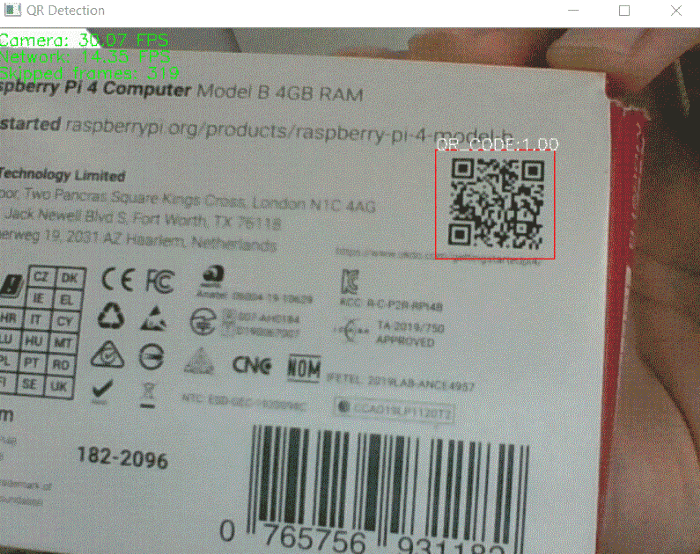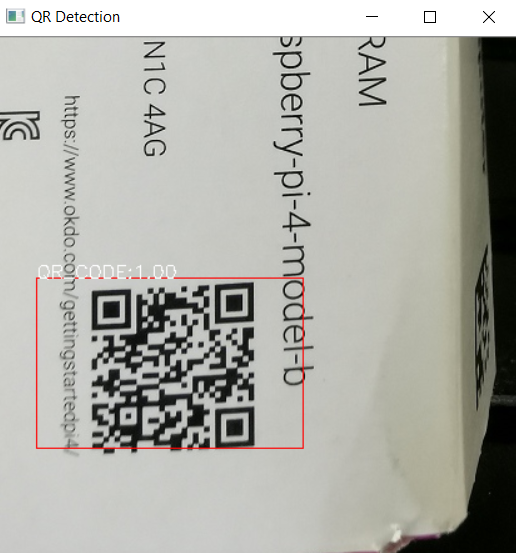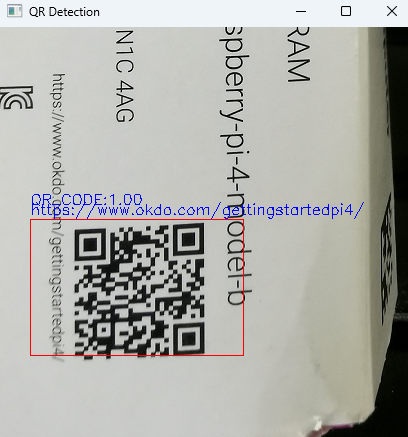# How to Detect and Decode QR Code with YOLO, OpenCV, and Dynamsoft Barcode Reader

In the past two weeks, I trained a custom YOLOv3 model for QR code detection and tested it with Darknet. In this article, I will use OpenCV’s DNN (Deep Neural Network) module to load the YOLO model for making detection from static images and real-time camera video stream. Besides, I will use Dynamsoft Barcode Reader to decode QR codes from the regions detected by YOLO.## YOLO QR Code Detection with OpenCV Python

Install OpenCV ( CPU only) via pip:

``````pip install opencv-python
``````

To quickly get familiar with the OpenCV DNN APIs, we can refer to object_detection.py, which is a sample included in the OpenCV GitHub repository.

### Implement the QR detection code logic step by step

First, we need to read an image to a Mat object using the `imread()` function. In case the image size is too large to display, we define the maximum width and height values:

``````frame = cv.imread("416x416.jpg")
threshold = 0.6
maxWidth = 1280; maxHeight = 720
imgHeight, imgWidth = frame.shape[:2]
hScale = 1; wScale = 1
thickness = 1
if imgHeight > maxHeight:
hScale = imgHeight / maxHeight
thickness = 6
if imgWidth > maxWidth:
wScale = imgWidth / maxWidth
thickness = 6
``````

The next step is to initialize the network by loading the `*.names`, `*.cfg` and `*.weights` files:

``````classes = open('qrcode.names').read().strip().split('\n')
net.setPreferableBackend(cv.dnn.DNN_BACKEND_OPENCV)
``````

The network requires a blob object as the input, therefore we can convert the Mat object to a blob object as follows:

``````blob = cv.dnn.blobFromImage(frame, 1/255, (416, 416), swapRB=True, crop=False)
``````

Afterwards, we input the blob object to the network to do inference:

``````ln = net.getLayerNames()
ln = [ln[i - 1] for i in net.getUnconnectedOutLayers()]
net.setInput(blob)
outs = net.forward(ln)
``````

As we get the network outputs, we can extract class names, confidence scores, and bounding boxes. In the meantime, we can draw them with OpenCV APIs:

``````def postprocess(frame, outs):
frameHeight, frameWidth = frame.shape[:2]

classIds = []
confidences = []
boxes = []

for out in outs:
for detection in out:
scores = detection[5:]
classId = np.argmax(scores)
confidence = scores[classId]
if confidence > threshold:
x, y, width, height = detection[:4] * np.array([frameWidth, frameHeight, frameWidth, frameHeight])
left = int(x - width / 2)
top = int(y - height / 2)
classIds.append(classId)
confidences.append(float(confidence))
boxes.append([left, top, int(width), int(height)])

indices = cv.dnn.NMSBoxes(boxes, confidences, threshold, threshold - 0.1)
for i in indices:
i = i
box = boxes[i]
left = box
top = box
width = box
height = box

# Draw bounding box for objects
cv.rectangle(frame, (left, top), (left + width, top + height), (0, 0, 255), thickness)

# Draw class name and confidence
label = '%s:%.2f' % (classes[classIds[i]], confidences[i])
cv.putText(frame, label, (left, top), cv.FONT_HERSHEY_SIMPLEX, 0.5, (255,255,255))

``````

Finally, we could adjust the image size to display appropriately on screen:

``````if hScale > wScale:
frame = cv.resize(frame, (int(imgWidth / hScale), maxHeight))
elif hScale < wScale:
frame = cv.resize(frame, (maxWidth, int(imgHeight / wScale)))
cv.imshow('QR Detection', frame)
cv.waitKey()
``````## Decoding QR Code with Dynamsoft Barcode Reader

Once the QR code detection is done, we can get the corresponding bounding boxes, with which we are able to take a further step to decode the QR code.

``````pip install dbr
``````

According to the coordinates of the bounding boxes, we can decode the QR code by setting the region parameters. The region decoding is much faster than the full image decoding:

``````from dbr import *

def decodeframe(frame, left, top, right, bottom):
settings.region_bottom  = bottom
settings.region_left    = left
settings.region_right   = right
settings.region_top     = top
settings.barcode_format_ids = EnumBarcodeFormat.BF_QR_CODE
settings.expected_barcodes_count = 1

try:
if text_results != None:
return text_results
print(bre)

return None
``````

On my screenshot, you can see the decoding result is obfuscated because I didn’t use a valid license key. If you want to experience the full functionalities of Dynamsoft Barcode Reader, you’d better apply for a free trial license to activate the Python barcode SDK.## Source Code

https://github.com/yushulx/opencv-yolo-qr-detection